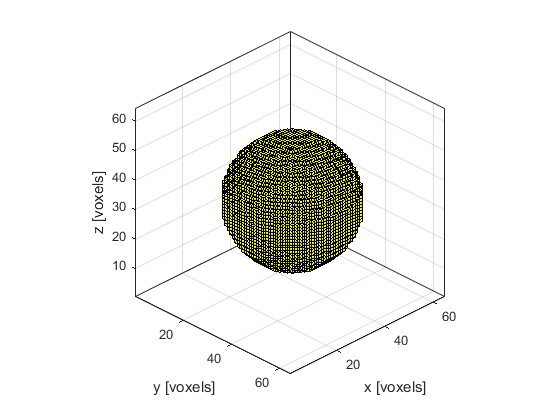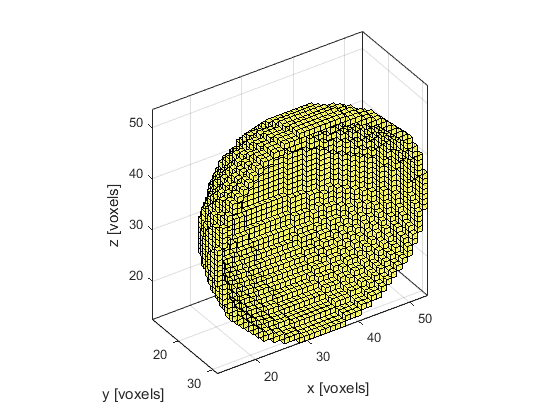# makeSphere

Create a binary map of a sphere within a 3D grid.

## Syntax

```sphere = makeSphere(Nx, Ny, Nz, radius)
sphere = makeSphere(Nx, Ny, Nz, radius, plot_sphere)
sphere = makeSphere(Nx, Ny, Nz, radius, plot_sphere, binary)
sphere = makeSphere(Nx, Ny, Nz, radius, [], binary)
```

## Description

`makeSphere` creates a binary map of a spherical shell (using an extension of the midpoint circle algorithm) within a three-dimensional grid. The sphere position is denoted by 1's in the matrix with 0's elsewhere. If the Boolean input parameter `binary` is set to `false` (the default), the sphere map is returned as a double precision matrix. If it is set to `true`, the map is returned as a logical matrix. A visualisation of the 3D grid returned by calling `makeSphere(64, 64, 64, 20, true);` and a cut away showing the singel grid point thickness (for illustrative purposes only) are given below.## Inputs

 `Nx, Ny, Nz` size of the 3D grid [grid points] `radius` sphere radius [grid points]

## Optional Inputs

 `plot_sphere` Boolean controlling whether the sphere is plotted using `voxelPlot` (default = `false`) `binary` Boolean controlling whether the sphere map is returned as a double precision matrix (`false`) or a logical matrix (`true`) (default = `false`)

## Outputs

 `sphere` 3D binary map of a sphere

## Examples

`makeBall`, `makeCartSphere`, `makeCircle`, `makeSphericalSection`